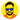# JS one-liners 😻

March 01, 2019🍵 4 min

I am a huge fan of Arrow functions. They have changed the way we write code. They have cured the cloudy behaviour of `this`. They let us define functions without `function` keyword 😅. This article collates one-liners — written using arrow functions — with their explanations.

### Remove Duplicates from Array

Interviewer often asks candidates to write a function which removes duplicates from an array. This can be done in many ways. We’ll discuss only those which are relevant to this post.

``````const removeDuplicates = arr =>
arr.filter((item, index) => index === arr.indexOf(item));``````

As you can see we are using `filter` and `indexOf` methods of the Array class. We get the index of the `item` using `indexOf` method and compare it with the current index. If both are equal then the `item` is unique else its repeated.

To remove duplicates we can also use Set data structures. We create a `set` from the `array` and then convert set back to the `array` using spread operator or `Array.from` method.

``````// Using spread operator.
const removeDuplicates1 = array => [...new Set(array)];
// Using Array.from method
const removeDuplicates2 = array => Array.from(new Set(array));``````

### Flatten Array

Flattening an array means converting a multi-dimension array to one-dimensional array. eg.

``````// 2-dimensional array.
[[1, 2, 3], [4, 5, 6]]
// After flattening
[1, 2, 3, 4, 5, 6]
// Multi-dimentional array
[1, [2, [3, 4, , 6], 7], 8], 9]
// After flattening
[1, 2, 3, 4, 5, 6, 7, 8, 9]``````

We can use `Array.concat` method with spread operator to flatten an array.

``````// Flattens an array(doesn't flatten deeply).
const flatten = array => [].concat(...array);``````

This approach flattens an array at only one level. Below function fully flattens an array.

``````const flattenDeep = arr =>
arr.reduce(
(fArr, item) => fArr.concat(Array.isArray(item) ? flatten(item) : item),
[]
);``````

### Merge Arrays

To merge arrays we can use `Array.concat` method with spread operator.

``````// Merge arrays
const merge = (...arrays) => [].concat(...arrays);``````

### Pipe (Function Composition)

We often need to “pipe” the output of one function as an input to another function. Here’s a simple implementation of the pipe function using Function Composition.

``````// Pipe fn
const pipe = (...fns) => arg => fns.reduce((v, fn) => fn(v), arg);

// Example
const addTwo = x => x + 2;
const double = x => x * 2;
const square = x => x * x;
const fn = pipe(addTwo, double, square);

### Compose(Pipe reverse)

Just like the `pipe` function, `compose` also supplies the output of one function as an input to another but it does it in reverse order.

``````const compose = (...fns) => fns.reduce((a, b) => (...args) => a(b(...args)));

// Example
const addTwo = x => x + 2;
const double = x => x * 2;
const square = x => x * x;
const fn = compose(addTwo, double, square);

### Range Function

You might have used `range` function of Lodash. Here’s a one-liner implementation of range using `Array.from` method. It generate range from `start` to `end` (exclusive). It also takes an optional `step` parameter.

``````// Generates range [start, end) with step
const range = (start, end, step = 1) =>
Array.from(
{ length: Math.ceil((end - start) / step) },
(_, i) => start + i * step
);``````

### Color Function

Generates a random hex color code.

``````// Generates random hex color code.
const color = () =>
'#' +
Math.floor(Math.random() * 0xffffff)
.toString(16)

### Conclusion

Before we conclude there’s one thing that should be kept it mind while writing one-liners. One-liners do more things with less code, which tempts people to write more one-liners. But sometimes, writing less code makes it less readable and hard to debug. So if you are writing code which you feel should or can be broken into multiple lines. Just go ahead and do it. You’ll thank yourself later 🙂.

One liners are great but one should always make sure that they don’t take a toll on the legibility of code.

Here’s the gist of all the one-liners used in this post. If there’s any one-liner which should be added to the list, please feel free to share. I’ll add it. Thanks for Reading 🙏🏻.Hi, I am Hitesh.

|